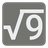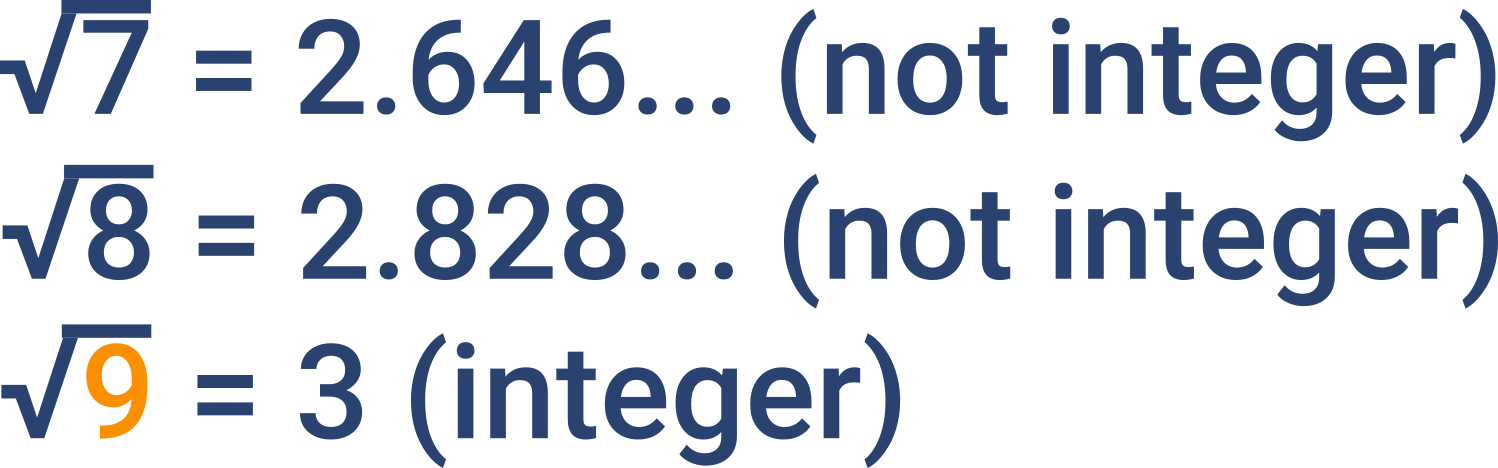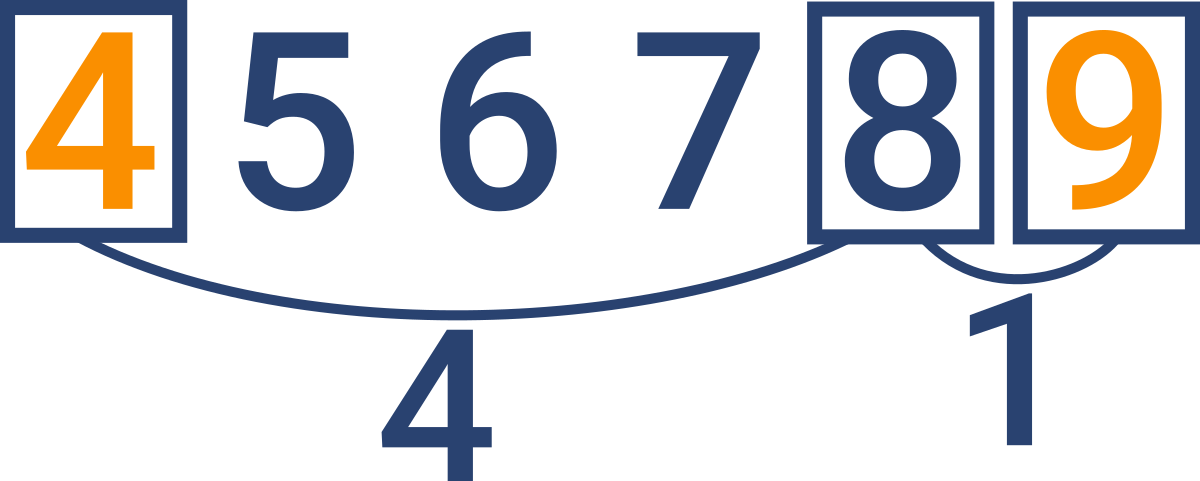The Nearest Square Number
Elementary+
English RU

You have some number and you are trying to find the nearest square number (a perfect square). Square number is the number the square root of which is an integer. For example, if we start with the number 8, then the two nearby square numbers are 4 (sqrt(4) == 2) and 9 (sqrt(9) == 3). So the answer is 9, because 9 is the nearest.Input: A number.

Output: The nearest square number.

Example:

```nearest_square(8) == 9
nearest_square(13) == 16
nearest_square(24) == 25
nearest_square(9876) == 9801```

How it is used: For mathematical analysis.

Precondition :
0 < number <= 1000000

Settings
Code:
CheckiO Extensions

CheckiO Extensions allow you to use local files to solve missions. More info in a blog post.

In order to install CheckiO client you'll need installed Python (version at least 3.8)

Install CheckiO Client first:

`pip3 install checkio_client`

`checkio --domain=py config --key=`

Sync solutions into your local folder

`checkio sync`

(in beta testing) Launch local server so your browser can use it and sync solution between local file end extension on the fly. (doesn't work for safari)

`checkio serv -d`

Alternatevly, you can install Chrome extension or FF addon

`checkio install-plugin`
`checkio install-plugin --ff`
`checkio install-plugin --chromium`

Read more here about other functionality that the checkio client provides. Feel free to submit an issue in case of any difficulties.

Sync...